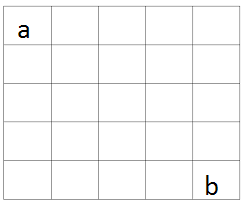# That's A Square

Probability Level 2

Given the following figure. if $a=5$ then find $b$ given that the number in the block is the arithmetic mean of the numbers in adjacent block.×